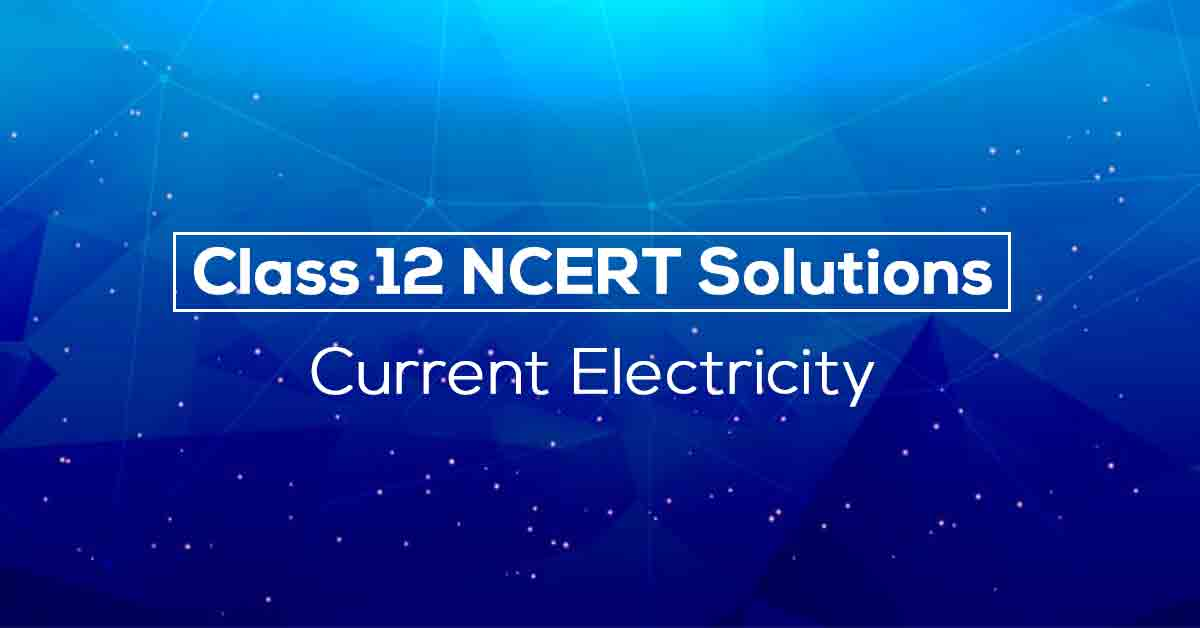Class 12th Physics Current Electricity NCERT Solution CBSE 2023

# NCERT Solutions for Class 12 Physics Chapter 3## NCERT Solutions for Class 12 Physics Chapter 3 Current Electricity – PDF Download

Vidyakul presents NCERT Solutions for Class 12 Physics Chapter 3 for students who want to learn fast and score more in the examination. These NCERT Solutions for Class 12 Physics Chapter 3 answers all the questions mentioned in Chapter-Current Electricity of NCERT Textbook. The NCERT Solutions for Class 12 Physics Chapter 3 pdf also contains notes which would help you understand the basic concepts in a simple yet effective way.

Current Electricity is an essential chapter in class 12 Physics, both from the board’s perspective as well as competitive exam’s perspective. The NCERT Solutions for Class 12 Physics Chapter 3 PDF covers the entire CBSE Physics syllabus and solutions to in text questions as well. Thus students are advised to follow these solutions and notes as not only they will help them to achieve more marks, but could also be used as the answer key to complete an assignment or homework faster.

Given below is an overview of the topics covered in NCERT Solutions for Class 12 Physics Chapter 3 PDF:

• ELECTRIC CURRENT
• ELECTRIC CURRENTS IN CONDUCTORS
• OHM’S LAW
• DRIFT OF ELECTRONS AND THE ORIGIN OF RESISTIVITY
• LIMITATIONS OF OHM’S LAW
• RESISTIVITY OF VARIOUS MATERIALS
• TEMPERATURE DEPENDENCE OF RESISTIVITY
• ELECTRICAL ENERGY, POWER
• COMBINATION OF RESISTORS – SERIES AND PARALLEL
• CELLS, EMF, INTERNAL RESISTANCE
• CELLS IN SERIES AND IN PARALLEL
• KIRCHHOFF’S RULES
• WHEATSTONE BRIDGE
• METER BRIDGE

Electrical Current is the flow of charged particles. The flow of charges will be constant in current electricity. For the current to flow we need a circuit. The electrons in a current flow from negative to positive. Electrons flow in the direction which is opposite to the direction of electric current.Know More about this in NCERT Solutions Class 12 Physics Chapter 3 PDF.

Download the FREE PDF of Physics Class 12 NCERT Solutions Chapter 3-Current Electricity and start your preparation with Vidyakul!